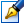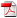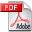##Cubic systems of differential equations with two and three invariant straight lines of maximal multiplicity

 Author: Vacaraş Olga Degree: doctor of physics and mathematics Speciality: 01.01.02 - Differential equations Year: 2018 Scientific advisers: Alexandru Şubădoctor habilitat, professor, Tiraspol State UniversityRomanovski Valery doctor habilitat ın ştiinţe fizico-matematice, profesor universitar, Slovenia Institution: Institute of Mathematics and Computer Science

### Status

The thesis was presented on the 19 May, 2017
Approved by NCAA on the 11 May, 2018

### Abstract0.57 Mb / in romanian

### Thesis

CZU 517.9251.02 Mb / in romanian
149 pages

### Keywords

cubic differential system, invariant straight line, multiplicity of an algebraic invariant curve, perturbed system, Darboux integrability.

### Summary

Field of study: qualitative theory of differential equations. The sub ject of study is the cubic system of differential equations with real coefficients.

The purpose and ob jectives: establishing the maximal multiplicity of an invariant straight line for differential polynomial systems; to give a classification of cubic systems with one, with two and with three invariant straight lines of maximal multiplicity; studying the problem of Darboux integrability for the obtained systems.

Scientific novelty and originality consists in the study of cubic systems of differential equations with non-degenerate infinity, having at most three multiple invariant straight lines (including the line at infinity) and in the establishing the maximal multiplicity of an invariant straight line for cubic systems and in the estimating the maximal algebraic multiplicity of an invariant straight line for polynomial systems of degree n, n ≥ 2.

The important scientific problem solved consists in the classification of cubic systems with one (the line at infinity), with two and with three invariant straight lines of maximal multiplicity and the construction of the perturbed cubic systems corresponding to the canonical forms in the case of the real invariant straight lines.

The theoretical significance: the obtained results in this thesis are new and are a continuation of the study of the cubic systems with invariant straight lines.

Implementation of the scientific results: the results of this thesis can be used: in the further investigations of cubic systems with invariant algebraic curves, as a support for teaching optional courses in higher education, in the study of some mathematical models which describe processes in physics, chemistry, biology, economy and others.